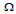# Digital Electronics - Multivibrators and 555 Timer

Exercise : Multivibrators and 555 Timer - General Questions
1.
What is the function of the comparators in the 555 timer circuit?
to compare the output voltages to the internal voltage divider
to compare the input voltages to the internal voltage divider
to compare the output voltages to the external voltage divider
to compare the input voltages to the external voltage divider
Explanation:
No answer description is available. Let's discuss.

2.
When a capacitor charges:
the voltage across the plates rises exponentially
the circuit current falls exponentially
the capacitor charges to the source voltage in 5×RC seconds
all of the above
Explanation:
No answer description is available. Let's discuss.

3.
The ________ is defined as the time the output is active divided by the total period of the output signal.
on time
off time
duty cycle
active ratio
Explanation:
No answer description is available. Let's discuss.

4.
A 22-kresistor and a 0.02-F capacitor are connected in series to a 5-V source. How long will it take the capacitor to charge to 3.4 V?
0.44 ms
0.501 ms
0.66 ms
0.70 ms